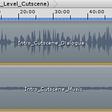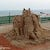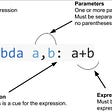# Get current timestamp using Python

A timestamp is a sequence of characters or encoded information used to find when a particular event occurred, generally giving the date and time of the day, accurate to a small fraction of a second. In this article, we will learn how to Get current timestamp in Python.

There are different ways to get current timestamp in Python, We can use functions from modules time, datetime and calendar.

1. Using module time :
The time module provides various time-related functions. The function time, return the time in seconds since the epoch as a floating point number. epoch is defined as the point where the time starts and is platform dependent.

`Syntax: time.time()Parameters: NAReturn: floating point number expressed in seconds.`

`# using time module`

`import` `time`

`# ts stores the time in seconds`

`ts =` `time.time()`

`# print the current timestamp`

`print(ts)`

Output:

`1594819641.9622827`

2. Using module datetime :
The datetime module provides classes for manipulating dates and times.
While date and time arithmetic is supported, the target of the implementation is on efficient attribute extraction for output formatting and manipulation. The function datetime.datetime.now which return number of seconds since the epoch.

`Syntax: datetime.now()Parameters: tz (time zone) which is optional.Return: the current local date and time.`

`# using datetime module`

`import` `datetime;`

`# ct stores current time`

`ct =` `datetime.datetime.now()`

`print("current time:-", ct)`

`# ts store timestamp of current time`

`ts =` `ct.timestamp()`

`print("timestamp:-", ts)`

Output:

`current time:- 2020-07-15 14:30:26.159446timestamp:- 1594823426.159446`

3. Using module calendar :
We can also get timestamp by combining multiple functions from multiple modules. In this we we’ll use function calendar.timegm to convert tuple representing current time.

`Syntax: calendar.timegm(tuple)Parameters: takes a time tuple such as returned by the gmtime() function in the time module.Return: the corresponding Unix timestamp value.`

`# using calendar module`

`# using time module`

`import` `calendar;`

`import` `time;`

`# gmt stores current gmtime`

`gmt =` `time.gmtime()`

`print("gmt:-", gmt)`

`# ts stores timestamp`

`ts =` `calendar.timegm(gmt)`

`print("timestamp:-", ts)`

Output:

gmt:- time.struct_time(tm_year=2020, tm_mon=7, tm_mday=15, tm_hour=19, tm_min=21, tm_sec=6, tm_wday=2, tm_yday=197, tm_isdst=0)
timestamp:- 1594840866

--

--

--

## More from Rakesh Tripathi

Love podcasts or audiobooks? Learn on the go with our new app.

## Software development life-cycle (SDLC)## Future of the FreeStuff Bot## Measure twice, cut once!## Text to SQL Queries## Rewriting a financial product using GraphQL## HOW TO INSTALL WORDPRESS## Lining up Audio & Visual to Build Cutscenes in Unity## Lambda Function in Python.